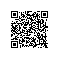# LeetCode 28：实现strStr() Implement strStr()

Implement strStr().

Return the index of the first occurrence of needle in haystack, or -1 if needle is not part of haystack.

Example 1:

Input: haystack = "hello", needle = "ll"Output: 2

Example 2:

Input: haystack = "aaaaa", needle = "bba"Output: -1

Clarification:

What should we return when needle is an empty string? This is a great question to ask during an interview.

For the purpose of this problem, we will return 0 when needle is an empty string. This is consistent to C's strstr() and Java's indexOf()).

needle 是空字符串时，我们应当返回什么值呢？这是一个在面试中很好的问题。

### 解题思路(Java):

• ##### 暴力穷举：
复杂度：时间 O(n^2) 空间 O(1)

字符串 a 从第一个索引开始 逐一匹配字符串 b 的第一个索引：a[i++]==b，如果为true，则进入内循环字符串a从第 i+j 个字符开始与字符串b 第 j个字符匹配：a[i+j]==b[j]

##### 代码：

class Solution { public int strStr(String haystack, String needle) { if(needle.equals(""))return 0; int haystackLen=haystack.length(),needleLen=needle.length(); char firstChar=needle.charAt(0);​ for(int i=0;i<=haystackLen-needleLen;i++){ if(haystack.charAt(i)==firstChar){ int j=1; for(;j if(haystack.charAt(i+j)!=needle.charAt(j)) break; } if(j==needleLen) return i; } } return -1; }}
• ##### KMP算法：
复杂度：时间 O(n+m) 空间 O(M)

下面引用一组图片帮助理解(图片来源：[https://blog.csdn.net/v_july_v/article/details/7041827](https://blog.csdn.net/v_july_v/article/details/7041827) )：

**说明：** 图片中字符串haystack为："BBC ABCDAB ABCDABCDABDE"，模式串 needle 为："ABCDABD"

> 第一步开始匹配：
>
>
> 第二步匹配到第一个相同字符：
>
>
> 第三步两个字符串逐一向后匹配，直到到字符 D 与 空格 字符匹配失败，结束该轮次匹配：
>
>
> 第四步重新匹配，但不用从第二步的下一个字符 B 开始，因为空格字符前与模式字符串前6个字符已经匹配相同。既C字符之前的两个字符 AB 与空格字符前两个字符 AB 相同，两个字符串可直接从 空白 字符与 C 字符开始匹配：
>

可以看到图片中一下跳过了 haystack 五个字符ABCDAB 和 needle 的两个字符AB。优化思路很清晰。

##### 代码：

class Solution { public int strStr(String haystack, String needle) { if(needle.equals("")) return 0; int[] next = new int[needle.length()]; getNext(next, needle);// 得到next数组 // i是匹配串haystack的指针，j是模式串needle的指针 int i = 0, j = 0; while(i < haystack.length() && j < needle.length()){ // 如果j=-1，即next数组中该字符为第一位，下标+1后，重新匹配 if(j == -1 || haystack.charAt(i) == needle.charAt(j)){ // 如果匹配成功，则自增1，匹配下一个字符 i++;j++; } else { j = next[j];// 如果匹配失败，则将j赋值next[j]，避免匹配重复匹配 } } return j == needle.length() ? i - j : -1; }​ private void getNext(int[] next, String needle){ // k是前缀中相同部分的末尾，也是相同部分的长度 // j是后缀的末尾，即后缀相同部分的末尾 int k = -1, j = 0; next = -1; while(j < needle.length() - 1){ // 如果k=-1，匹配失败，重新开始计算前缀和后缀相同的长度 // 如果两个字符相等，则在上次前缀和后缀相同的长度加1，继续下一段字符最大公共前后缀匹配 if (k == -1 || needle.charAt(j) == needle.charAt(k)){ k++;j++; if (needle.charAt(j) != needle.charAt(k)) next[j] = k; else //因为不能出现p[j] = p[ next[j ]]，所以当出现时需要继续递归，k = next[k] = next[next[k]]，以减少重复部分的多余匹配 next[j] = next[k]; } else { // 否则，前缀长度缩短为next[k] k = next[k]; } } }}

### 总结：

KMP算法优化的方向很明了，主要难点就在于对next数组的求法和理解，KMP算法不是本文的重点，如有兴趣深入了解，推荐一篇博文：https://blog.csdn.net/v_july_v/article/details/7041827

• 如果该字符没有在模式串中出现，直接从该字符向右移动位数 = 模式串长度 + 1。（因为源字符串含有该字符的相同长度字符串不可能匹配）

• 如果该字符在模式串中出现过，其移动位数 = 模式串中最右端的该字符到末尾的距离+1。

### Python3：

class Solution: def strStr(self, haystack: str, needle: str) -> int: return haystack.find(needle)
##### find() 方法描述

find() 方法检测字符串中是否包含子字符串 str ，如果指定 beg（开始） 和 end（结束） 范围，则检查是否包含在指定范围内，如果指定范围内如果包含指定索引值，返回的是索引值在字符串中的起始位置。如果不包含索引值，返回-1。如果子字符串为空，返回0。

##### 语法

str.find(str, beg=0, end=len(string))
##### 参数
• str -- 指定检索的字符串

• beg -- 开始索引，默认为0。

• end -- 结束索引，默认为字符串的长度。

class Solution: def strStr(self, haystack: str, needle: str) -> int: for i in range(len(haystack)-len(needle)+1): if haystack[i:i+len(needle)]==needle:#截取切片 return i return -1使用钉钉扫一扫加入圈子
+ 订阅